# Difference between revisions of "12-240/Classnotes for Thursday October 4"

## Reminders

Web Fact: No link, doesn't exist!

Life Fact: Dror doesn't do email math!

Riddle: Professor and lion in a ring with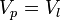$V_p = V_l$, help the professor live as long as possible.

## Recap

Base - what were doing today

Linear combination (lc) - We say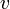$v$ is a linear combination of a set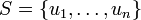$S = \{u_1, \dots, u_n\}$ if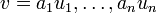$v = a_1u_1, \dots, a_nu_n$ for scalars from a field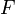$F$.

Span -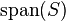$\operatorname{span}(S)$ is the set of all linear combinations of the set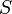$S$.

Generate - We say$S$ generates a vector space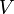$V$ is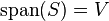$\operatorname{span}(S) = V$.

## Pre - Basis

Linear dependence

Definition A set S ⊂ V is called linearly dependent if you can express the zero vector as a linear combination of distinct vectors from S, excluding the non-trivial linear combination where all of the scalars are 0.

Otherwise, we call S linearly independent.

### Examples

1. In R^3, take S = {u1 = (1,4,7), u2 = (2,5,8), u3 = (3,6,9)}

u1 - 2u2 + u3 = 0

S is linearly dependent.

2. In R^n, take {e_i} = {0, 0, ... 1, 0, 0, 0} where 1 is in the ith position, and (ei) is a vector with n entries.

Claim: This set is linearly independent.

Proof: Suppose (∑ ai∙ei) = 0 ({ei} is linearly dependent.)

(∑ ai∙ei) = 0 ⇔ a1(1, 0, ..., 0) + ... + an(0, ..., 0, 1) = 0 ⇔ (a1, 0, ... , 0) + ... + (0, ... , an) = 0

⇒ a1 = a2 = ... = an = 0!

1. {u} is linearly independent. Proof: ⇐ If u≠0, suppose au =0 By property (a*u = 0, a = 0 or u = 0), a = 0. Thus, {u} is linearly independent.

⇒ By definition, au = 0 for {u} only when a = 0.

2. ∅ is linearly independent.

Exercise: Prove: Theorem Suppose S1 ⊂ S2 ⊂ V.

-> If S1 is linearly dependent, then S2 is dependent.

-> If S2 is linearly independent, then S1 is linearly independent. (Hint: contrapositive)

## Basis

Definition: A subset β is called a basis if 1. β generates V → span(β) = V and 2. β is linearly independent.

### Examples

1. V = {0}, β = {}

2. {ei} for F^n, this is what we call the standard basis

3. B = {(1,1),(1, -1)} is a basis for R^2

4. P_n(F) β = {x^n, x^n-1, ... , x^1, x^0}

5. P(F), β = (x^0, x^1 ... and on} (Infinite basis!)

## Interesting inequality

This holds is true if the field does not have characteristic 2. Can you see why?

(a,b) = (a+b)/2 * (1, 1) + (a-b)/2 * (1, -1)

[/itex]